# Edges and Corners

## Edges

Edges are defined by single lines:

e(x, y) = ax + by + c The edge image is defined by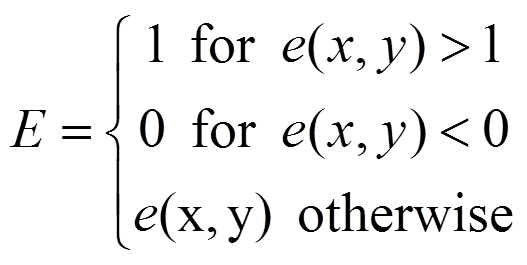The figure below shows a generated edge image: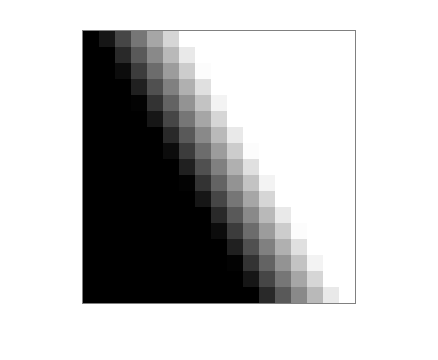See MATLAB code that generated this image.

## Corners

Corners are defined by two intersecting lines:represented graphically by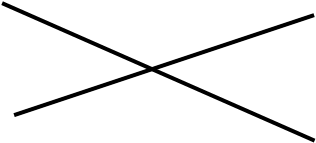The edge function is given by the product of the lines, and can be reduced to a general quadratic as shown below.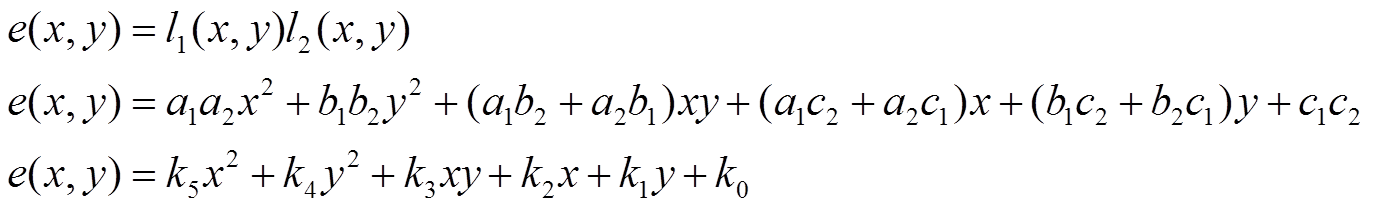If the lines are perpendicular, the corresponding vectors must have a zero dot product, as shown below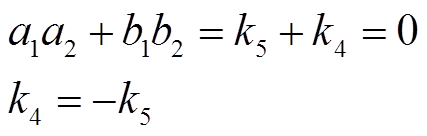This eliminates a term proportional to x2 + y2.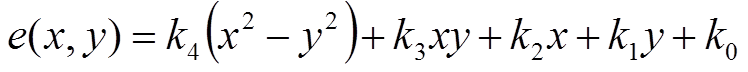The figure below shows a generated corner image determined by xy: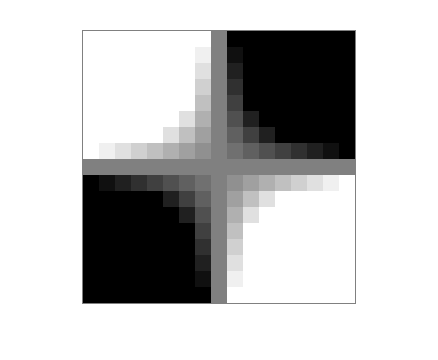See MATLAB code that generated this image.

## Examples

### Example 1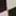pixels in the right-hand image above are interpolated

### Example 2pixels in the right-hand image above are replicated

Maintained by John Loomis, last updated 1 November 2013